# An Introduction to the nullabor package

## Introduction

``````library(nullabor)
library(ggplot2)
library(dplyr)
``````

The nullabor package provides functions to support visual inference, as described in the papers:

Buja, A., Cook, D., Hofmann, H., Lawrence, M., Lee, E.-K., Swayne, D. F, Wickham, H. (2009) Statistical Inference for Exploratory Data Analysis and Model Diagnostics, Royal Society Philosophical Transactions A, 367:4361–4383.

Wickham, H., Cook, D., Hofmann, H. and Buja, A. (2010) Graphical Inference for Infovis, IEEE Transactions on Visualization and Computer Graphics, 16(6):973–979, http://doi.ieeecomputersociety.org/10.1109/TVCG.2010.161. Best paper award.

Hofmann, H., Follett, L., Majumder, M. and Cook, D. (2012) Graphical Tests for Power Comparison of Competing Designs, IEEE Transactions on Visualization and Computer Graphics, 18(12):2441–2448, http://doi.ieeecomputersociety.org/10.1109/TVCG.2012.230.

Majumder, M., Hofmann, H. and Cook, D. (2013) Validation of Visual Statistical Inference, Applied to Linear Models, Journal of the American Statistical Association, 108(503):942–956. Featured Article http://amstat.tandfonline.com/doi/pdf/10.1080/01621459.2013.808157.

Roy Chowdhury, N., Cook, D., Hofmann, H., Majumder, M., Lee, E. K., Toth, A. (2014) Understanding High Dimension, Small Sample Size Problems Using Visual Statistical Inference, Computational Statistics, To appear.

Majumder, M. and Hofmann, H. and Cook, D. (2014) Human Factors Influencing Visual Statistical Inference, http://arxiv.org/abs/1408.1974.

Roy Chowdhury, N. and Cook, D. and Hofmann, H. and Majumder, M. and Zhao, Y. (2014) Utilizing Distance Metrics on Lineups to Examine What People Read From Data Plots, http://arxiv.org/abs/1408.1889.

The functions provide methods for two protocols: lineup and rorschach. The lineup protocol places the plot of the actual data among a field of plots of null data, and in the Rorschach, all plots are of null data. The encrypt function enables the location of the actual data plot to be a secret, that needs decrypt to reveal. There are several different functions for generating null data sets: null_permute, null_lm and null_dist.

The functions reg_dist, bin_dist, uni_dist, box_dist, sep_dist are ways of calculating how different one plot is from another. These are used to get a sense if the actual data plot is different from the null plots, in as far as we can determine numerically. The functions distmet and distplot compute a rough estimate of the distribution of the distance measures for the data and the null generating mechanism, and make a plot where the values for the actual data plot and the null plots in a lineup are shown. This helps a little to evaluate whether people should easily pick the actual data plot from the lineup and thus help to organize the Amazon Turk experiments (http://www.public.iastate.edu/~hofmann/experiments.html)[http://www.public.iastate.edu/~hofmann/experiments.html] some.

## The lineup protocol

In this protocol, the plot of the real data is randomly embedded amongst a set of null plots. The matrix of plots is known as a lineup. The null plots are generated by a method consistent with the null hypothesis. The lineup is shown to an observer. If the observer can pick the real data as different from the others, this puts weight on the statistical significance of the structure in the plot. The `"lineup"` function returns a set of generated null datasets and the real data embedded randomly among these null datasets. The method of null generation should be provided in the lineup function for the null datasets to be generated automatically along with the real dataset. The users also have the option of generating the null datasets themselves and providing them in the `"lineup"` function. The position of the real dataset can be left missing and the function picks the position at random. The function then returns the position as an encrypted code. The encrypted code is copied and pasted on the console to obtain the true position of the plot.

``````d <- lineup(null_permute("mpg"), mtcars)
``````
``````## decrypt("C73P tY1Y nW lZOn1nZW c")
``````
``````head(d)
``````
``````##    mpg cyl disp  hp drat    wt  qsec vs am gear carb .sample
## 1 22.8   6  160 110 3.90 2.620 16.46  0  1    4    4       1
## 2 18.1   6  160 110 3.90 2.875 17.02  0  1    4    4       1
## 3 33.9   4  108  93 3.85 2.320 18.61  1  1    4    1       1
## 4 30.4   6  258 110 3.08 3.215 19.44  1  0    3    1       1
## 5 13.3   8  360 175 3.15 3.440 17.02  0  0    3    2       1
## 6 21.4   6  225 105 2.76 3.460 20.22  1  0    3    1       1
``````
``````# Position of actual data plot
attr(d, "pos")
``````
``````##  7
``````

The lineup data can be then used to generate the lineup using ggplot2. The lineup is shown to one or more observers who are asked to identify the plot which is different. If the observer can identify the plot of the real data correctly, we reject the null hypothesis and conclude that the plot of the real data has stronger structure than the null plots.

``````qplot(mpg, wt, data = d) + facet_wrap(~ .sample)
``````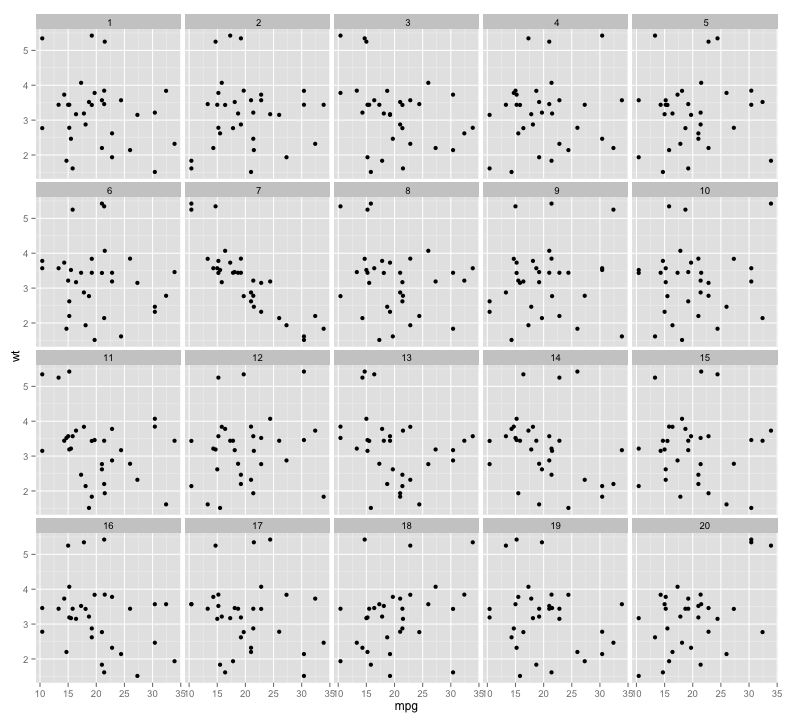## The Rorschach protocol

The Rorschach protocol is used to calibrate the eyes for variation due to sampling. The plots generated corresponds to the null datasets, data that is consistent with a null hypothesis. The `"rorschach"` function returns a set of null plots which are shown to observers to calibrate their eyes with variation. Like the `"lineup"` function, the null generating mechanism should be provided as an input along with a real dataset. A probability can also be given as input which dictates the chance of including the true data with null data.

``````d <- rorschach(null_permute("mpg"), mtcars, n = 20, p = 0)
qplot(mpg, wt, data = d) + facet_wrap(~ .n)
``````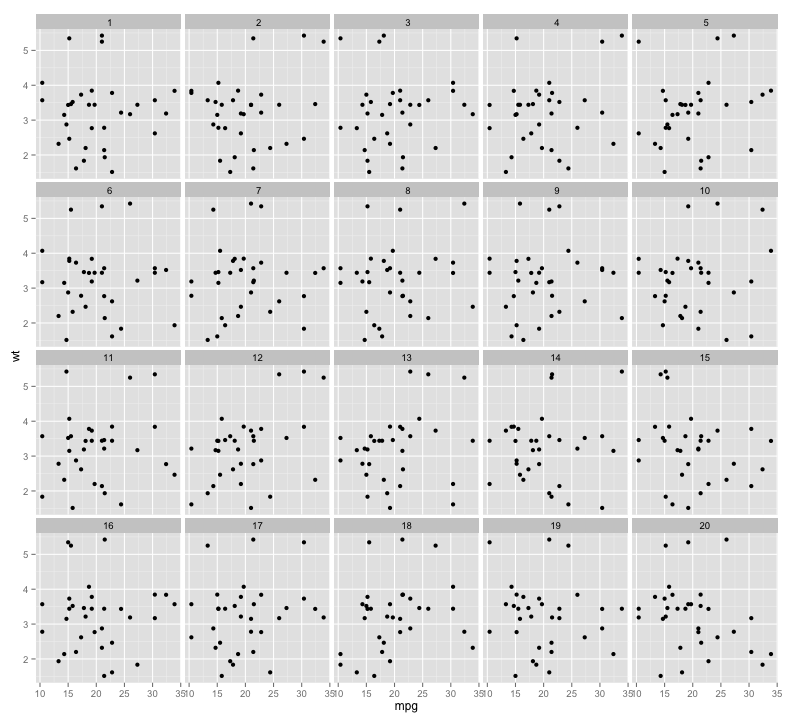## Generate null data with a specific distribution

The `"null_dist"` function takes as input a variable name of the data and a particular distribution. This variable in the data is substituted by random generations of the particular distribution. The different distributions include beta, cauchy, chi-squared, exponential, f, gamma, geometric, log-normal, lognormal, logistic, negative binomial, normal, poisson, t and weibull. A list of parameters of distribution can also be provided as input. In case it is not provided, `"fitdistr"` is used to estimate the parameters from the given data. The function `"null_dist"` returns a function that given the data generates a null data set.

``````head(null_dist("mpg", dist = "normal")(mtcars))
``````
``````##                     mpg cyl disp  hp drat    wt  qsec vs am gear carb
## Mazda RX4         23.49   6  160 110 3.90 2.620 16.46  0  1    4    4
## Mazda RX4 Wag     20.05   6  160 110 3.90 2.875 17.02  0  1    4    4
## Datsun 710        17.83   4  108  93 3.85 2.320 18.61  1  1    4    1
## Hornet 4 Drive    29.07   6  258 110 3.08 3.215 19.44  1  0    3    1
## Hornet Sportabout 21.65   8  360 175 3.15 3.440 17.02  0  0    3    2
## Valiant           25.99   6  225 105 2.76 3.460 20.22  1  0    3    1
``````

## Generate null data by permuting a variable

The `"null_permute"` function takes as input a variable name of the data. This variable is permuted to obtain the null dataset. The function `"null_dist"` returns a function that given the data generates a null data set.

``````head(null_permute("mpg")(mtcars))
``````
``````##                    mpg cyl disp  hp drat    wt  qsec vs am gear carb
## Mazda RX4         26.0   6  160 110 3.90 2.620 16.46  0  1    4    4
## Mazda RX4 Wag     30.4   6  160 110 3.90 2.875 17.02  0  1    4    4
## Datsun 710        22.8   4  108  93 3.85 2.320 18.61  1  1    4    1
## Hornet 4 Drive    21.4   6  258 110 3.08 3.215 19.44  1  0    3    1
## Hornet Sportabout 21.5   8  360 175 3.15 3.440 17.02  0  0    3    2
## Valiant           16.4   6  225 105 2.76 3.460 20.22  1  0    3    1
``````

## Generate null data with null residuals from a model

The function `"null_lm"` takes as input a model specification formula as defined by `"lm"` and method for generating null residuals from the model. The three built in methods are 'rotate', 'pboot' and 'boot' defined by `"resid_rotate"`, `"resid_pboot"` and `"resid_boot"` respectively. The function returns a function which given the data generates a null dataset.

``````head(null_lm(wt~mpg, method = 'rotate')(mtcars))
``````
``````##                    mpg cyl disp  hp drat    wt  qsec vs am gear carb
## Mazda RX4         21.0   6  160 110 3.90 3.420 16.46  0  1    4    4
## Mazda RX4 Wag     21.0   6  160 110 3.90 2.287 17.02  0  1    4    4
## Datsun 710        22.8   4  108  93 3.85 3.266 18.61  1  1    4    1
## Hornet 4 Drive    21.4   6  258 110 3.08 3.497 19.44  1  0    3    1
## Hornet Sportabout 18.7   8  360 175 3.15 3.563 17.02  0  0    3    2
## Valiant           18.1   6  225 105 2.76 3.932 20.22  1  0    3    1
##                    .resid .fitted
## Mazda RX4          0.3306   3.089
## Mazda RX4 Wag     -0.8022   3.089
## Datsun 710         0.4304   2.836
## Hornet 4 Drive     0.4641   3.033
## Valiant            0.4342   3.498
``````

## Distance metrics

There are five different distance metrics in nullabor package, named `"bin_dist"`, `"box_dist"`, `"reg_dist"`, `"sep_dist"` and `"uni_dist"`. The different distance metrics are constructed so that they can identify the different properties of the data. `"uni_dist"` works for univariate data while the others works for all types of bivariate data. Binned distance is a generic distance which can be used in any situations while the other distance metrics are constructed so that they can identify the effect of graphical elements in a plot like an overlaid regression line or presence of defined clusters. To calculate some of the metrics, additional informations like a class variable or the number of bins should be provided.

## Distance for univariate data

`"uni_dist"` is a distance metric which calculates the euclidean distance between the first four central moments of two univariate data. A typical usage would be when one needs to calculate the distance between the two histograms drawn from two datasets.

``````uni_dist(rnorm(100), rpois(100, 2))
``````
``````##  2.186
``````

## Distance based on regression parameters

`"reg_dist"` is a distance metric which calculates the euclidean distance between the regression parameters of a model fitted to one plot and that of another plot. It is advisable to use this distance in situations where a regression line is overlaid on a scatterplot.

``````with(mtcars, reg_dist(data.frame(wt, mpg), data.frame(sample(wt), mpg)))
``````
``````##  168.3
``````

## Distance based on boxplots

`"box_dist"` is a distance metric which works for side-by-side boxplots with two levels. The first quartile, median and the third quartile are calculated for each box and the absolute distances of these are calculated for the two boxes. `"box_dist"` calculates the euclidean distance between these absolute distances for the two plots. The boxplot distance should be used in situations where a side-by-side boxplot is used to compare the distribution of a variable at two different levels.

``````with(mtcars, box_dist(data.frame(as.factor(am), mpg),  data.frame(as.factor(sample(am)), mpg)))
``````
``````##  5.555
``````

## Distance based on separation

`"sep_dist"` is a distance metric based on the separation between clusters. The separation between clusters is defined by the minimum distances of a point in the cluster to a point in another cluster. The separation between the clusters for a given dataset is calculated. An euclidean distance is calculated between the separation for the given dataset and another dataset. The number of clusters in the dataset should be provided. If not, the hierarchical clustering method is used to obtain the clusters.

``````with(mtcars, sep_dist(data.frame(wt, mpg,  as.numeric(as.factor(mtcars\$cyl))), data.frame(sample(wt), mpg,  as.numeric(as.factor(mtcars\$cyl))), nclust = 3))
``````
``````##  0.03171
``````

## Binned Distance

`"bin_dist"` is a generic distance which works for any situation for any dataset. For a given bivariate dataset, X and Y variables are divided into p and q bins respectively to obtain pq cells. The number of points falling in each cell are counted for a given dataset. `"bin_dist"` between two datasets calculates the euclidean distance between the cell counts of these two data. The values of p and q should be provided as arguments.

``````with(mtcars, bin_dist(data.frame(wt, mpg), data.frame(sample(wt), mpg), lineup.dat = NULL, X.bin = 5, Y.bin = 5))
``````
``````##  10.39
``````

## Calculating the mean distances for the plots in the lineup

It is interesting to see whether the true plot in a lineup is different from all the null plots. To find this the distances between the true plot and all the null plots are calculated and the mean of these distances is calculated. Similarly, for each null plot, the distance between the null plot and all the other null plots is calculated and averaged to obtain the mean distance for each null plot. `"calc_mean_dist"` calculates the mean distance corresponding to each plot in the lineup. If the mean distance of the true plot is larger than the mean distances of all the null plots, the lineup is considered easy. If one of the null plots has a larger mean distance than the true plot, the lineup is considered difficult.

``````calc_mean_dist(lineup(null_permute('mpg'), mtcars, pos = 10), var = c('mpg', 'wt'), met = 'reg_dist', pos = 10)
``````
``````## Source: local data frame [20 x 2]
##
##    plotno mean.dist
## 1       1    0.8844
## 2       2    0.5357
## 3       3    0.3636
## 4       4    0.4043
## 5       5    2.2055
## 6       6    0.5270
## 7       7    0.3634
## 8       8    0.4269
## 9       9    0.3936
## 10     10    8.4595
## 11     11    0.4733
## 12     12    0.7039
## 13     13    1.0335
## 14     14    1.6593
## 15     15    0.3657
## 16     16    1.0045
## 17     17    1.2270
## 18     18    0.4038
## 19     19    0.3986
## 20     20    0.4108
``````

## Calculating difference measure for lineups

The mean distances for each plot in the lineup are obtained using `"calc_mean_dist"`.`"calc_diff"` calculates the difference between the mean distance for the true plot and the maximum mean distance for the null plots.

``````calc_diff(lineup(null_permute('mpg'), mtcars, pos = 10), var = c('mpg', 'wt'), met = 'reg_dist', dist.arg = NULL, pos = 10)
``````
``````##  7.745
``````

## Optimum number of bins

Binned distance is highly affected by the choice of the number of bins. The number of bins is provided by the user and this can be subjective. This motivates to design a way to select the optimum number of bins to be used. `"opt_diff"` finds the optimal number of bins in both x and y direction which should be used to calculate the binned distance. The binned distance is calculated for each combination of provided choices of number of bins in x and y direction and finds the difference using `"calc_diff"` for each combination. The combination for which the difference is maximum should be used.

``````opt.diff <- opt_bin_diff(lineup(null_permute('mpg'), mtcars, pos = 10), var = c('mpg', 'wt'), 2, 4, 2, 4, pos = 10, plot = TRUE)
opt.diff\$p
``````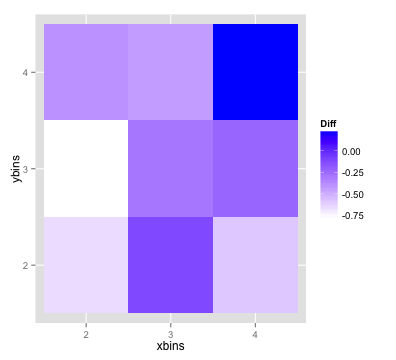## Distribution of distance metrics

Measuring the quality of a lineup is interesting. But it may also be important to compare a few lineups. The `"distmet"` function provides the empirical distribution of the distance metrics based on the mean distance of the true plot and the mean distance from the null plots. The lineup data, the null generating mechanism and the choice of the distance metric has to be provided. Users have the flexibility of using their distance metrics. The position of the true plot in the lineup has to be provided as well. If the distance metrics require additional arguments, those have to be provided as well.

``````lineup.dat <- lineup(null_permute('mpg'), mtcars, pos = 10)
qplot(mpg, wt, data = lineup.dat, geom = 'point') + facet_wrap(~ .sample)
``````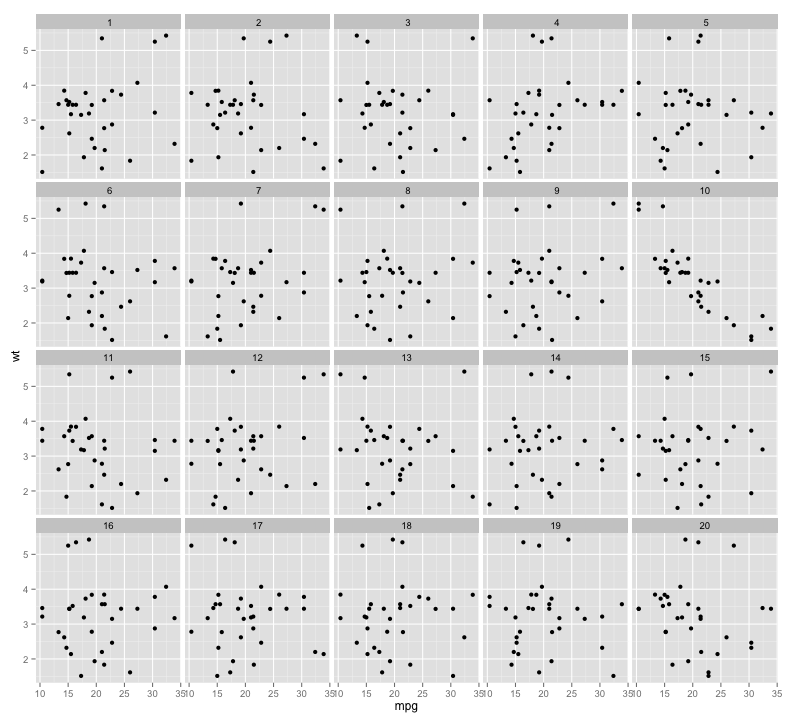Copy and paste the output of lineup.dat to get the position of the true plot

``````#decrypt('...')
# 'True data in position 10' # Use pos = 10
``````
``````dist.vals <- distmet(lineup.dat, var = c('mpg', 'wt'),'reg_dist', null_permute('mpg'), pos = 10, repl = 100, dist.arg = NULL)
``````
``````head(dist.vals\$lineup)
``````
``````## Source: local data frame [6 x 2]
##
##   plotno mean.dist
## 1      1    1.0057
## 2      2    0.5776
## 3      3    0.4076
## 4      4    1.4244
## 5      5    0.4379
## 6      6    1.0827
``````
``````dist.vals\$diff
``````
``````##  7.411
``````
``````head(dist.vals\$closest)
``````
``````##   7  4  6  1 12
``````
``````head(dist.vals\$null_values)
``````
``````##  0.3736 0.7324 0.3420 0.1470 0.4486 0.8789
``````
``````dist.vals\$pos
``````
``````##  10
``````
``````dist.vals <- distmet(lineup.dat, var = c('mpg', 'wt'),'bin_dist', null_permute('mpg'), pos = 10, repl = 100, dist.arg = list(lineup.dat = lineup.dat, X.bin = 5, Y.bin = 5))
``````

## Plotting the empirical distribution of the distance metric

`"distplot"` functions plots the empirical distribution of the distance metric, given the output of `"distmet"` function. The distribution is shown in grey along the distance for the true plot in orange and the distances for the null plots in black.

``````distplot(dist.vals)
``````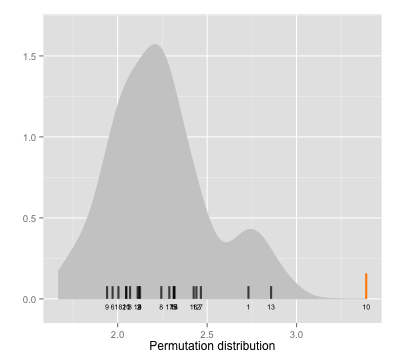h